Calculating Power Conversion Efficiencies for Direct and Non-Direct Sunlight Photovoltaics

Eden Rephaeli March 18, 2007

(Submitted as coursework for AP272, StanfordUniversity, Winter 2007)

In this report I shall describe two important models for solar power conversion. These models serve as important paradigms when thinking or designing solar power systems. The systems described are somewhat ideal but very instructive, and serve to establish an upper bound for efficiencies one might hope to attain. In both models the "cell" is taken to be a semiconducting single p-n junction device characterized by its energy band gap Eg.

Direct Sunlight Model 

We consider a simple system consisting of a planar p-n junction modeled as a blackbody at temperature Tc. The sun will be modeled as a blackbody at temperature Ts=6000K. In order to get an upper limit on the efficiency of such a system the following hypothesis is made : every photon incident on the cell with energy greater than Eg creates one electronic charge e at a voltage of Eg/e.

We begin our efficiency calculation by making a further simplification and considering a cell at a specific temperature, namely: Tc = 0 K, at this temperature the cell does not produce any blackbody radiation itself. The power incident on the cell is equal to the product of the sun's intensity and the area of the cell: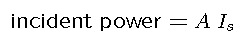Where A is the area of the cell and Is is given by the blackbody model  (energy per unit area per unit time):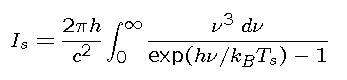In accordance with our initial hypothesis, the power output is the product of the number of photons incident on the cell per unit time with energy greater than the band gap, and the energy of each electronic excitation produced by each of these photons: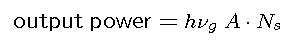where Ns is the number of photons with energy greater than the band gap per unit are per unit time: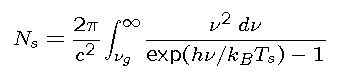The lower integral is carried out only over photon frequencies which are greater than or equal to the gap energy. The Ultimate Efficiency is simply given by the ratio of output power to incident power: (converting both integrals to a dimensionless form)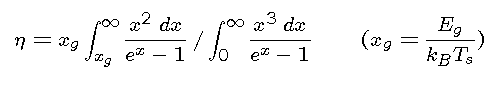It is apparent that the efficiency is solely a function of the variable xg. The efficiency is maximized by a cell with a band gap energy of 1.1 eV, where the efficiency is 44%. Missing in the above calculation are the following factors:

• A less than unity probability that an incident photon with energy equal to or greater than the band gap will create an electronic excitation.
• Radiative and non-radiative recombination of the electronic excitations before they are managed to be extracted from the cell.
• The fact that the cell is not maintained at 0K but at roughly 300K
• Load matching involved in maximizing the electrical power extracted from the cell.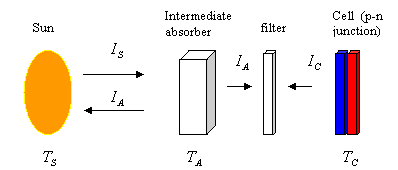Fig. 1: A schematic description of the indirect sunlight system.

When the last two factors on the list are accounted for, the efficiency goes down to 41%. Experimentally, the current upper efficiency limit for a single junction system considered here is roughly 30%, the first two factors in the above list are what account for the difference.

Indirect Sunlight Model 

It is possible to improve upon the efficiency of direct sunlight solar cells by placing an intermediate body between sun and cell (figure 1). In addition it is helpful to place a filter between the intermediate and cell. We shall consider a filter which transmits a narrow, nearly monochromatic spectrum at around the band gap energy. To complete the model, assume the intermediate to be a blackbody of temperature TA. In this case the efficiency will be the product of sun-absorber and absorber-cell power conversion efficiency.

Assuming full concentration  of the sun's radiation we write the sun's incident power per unit area on the intermediate: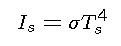where σ is the Stefan-Boltzmann constant. Note that this is just the second equation above integrated over all frequencies. The intermediate, being a black body emits an intensity of the same form but with temperature TA towards the sun. Therefore, the efficiency of power conversion between sun and intermediate is given by: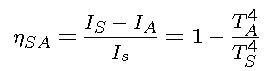Turning to the intermediate-cell side of the system, and "replacing" the sun with the intermediate we find that repeating the calculation of Eq. 5 would result in: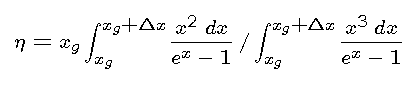where Δx represents the energy width of the filter. By letting the energy spectrum approach monochromatic we get 100% efficiency. This establishes the motivation for considering an intermediate. Therefore we must consider a more detailed The cell which is at temperature Tc generates blackbody radiation and therefore emits a number of photons per unit area equal to :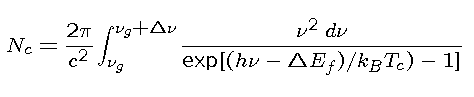where the limits of integration go from νg to νg + Δν are brought about by the filter.

Notice that the only difference between Eq. 4 and Eq. 9 is the introduction of ΔEf, which represents the difference in quasi Fermi levels (and the temperature of the body). The Fermi level of the cell is split due to the quasi equilibrium conditions which persist as a result of incident radiation from the intermediate. ΔEf is the chemical energy per electron hole pair created in the cell and ΔEf/e is the open circuit voltage created in the cell. The intermediate emits a number of photons per unit area equal to Eq. 4 but with temperature

In the above quasi equilibrium that exists, the number of photons per unit area incident on the cell must equal the number leaving the cell, which brings about the following equality: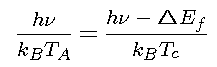The efficiency of energy conversion is equal to the ratio between the chemical energy per electron hole pair and the photon energy coming from the intermediate through the filter: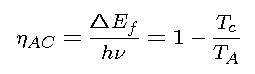The last equality is a result of using Eq. 10. We recognize the above as Carnot efficiency . In analogy to a Carnot process, the intermediate and cell can be thought of as the two heat baths and the work done is in exciting of electron hole pairs in the cell. It is clear from this analogy that this equality holds only as long as current is extracted from the cell, a process which would generate entropy and thus lower the efficiency. The composite efficiency is given by the product of Eq. 7 and Eq. 11: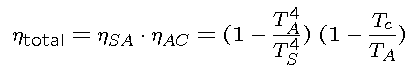Taking Tcs = 600 K the efficiency reaches a maximum of 85%. We may consider non idealities which are missing from this picture. They decrease the efficiency yet maintain the overall picture of potential for improvement over the direct sunlight model.

The above two models are important paradigms, but there are more subtleties and changes which can be introduced. However, these two models embody the thinking and reasoning behind all models, revealing important insight to the considerations underlying the physical design of photovoltaic systems.

© 2007 Eden Raphaeli. The author grants permission to copy, distribute and display this work in unaltered form, with attribution to the author, for noncommercial purposes only. All other rights, including commercial rights, are reserved to the author.

References:

 W. Shockley W and H. J. Queisser, J. Appl. Phys. 32, 510 (1961).

 M. Planck (1901), Ann. d. Phys. 4, 553 (1901).

 L. D. Landau and E. M. Lifshitz, Statistical Physics, Part 1 (Pergamon, 1980).

 N. P. Harder and P. Wurfel, Semicond. Sci. Technol. 18, S151 (2003).

 G. Lasher and F. Stern F, Phys. Rev. 133A, 553 (1964).